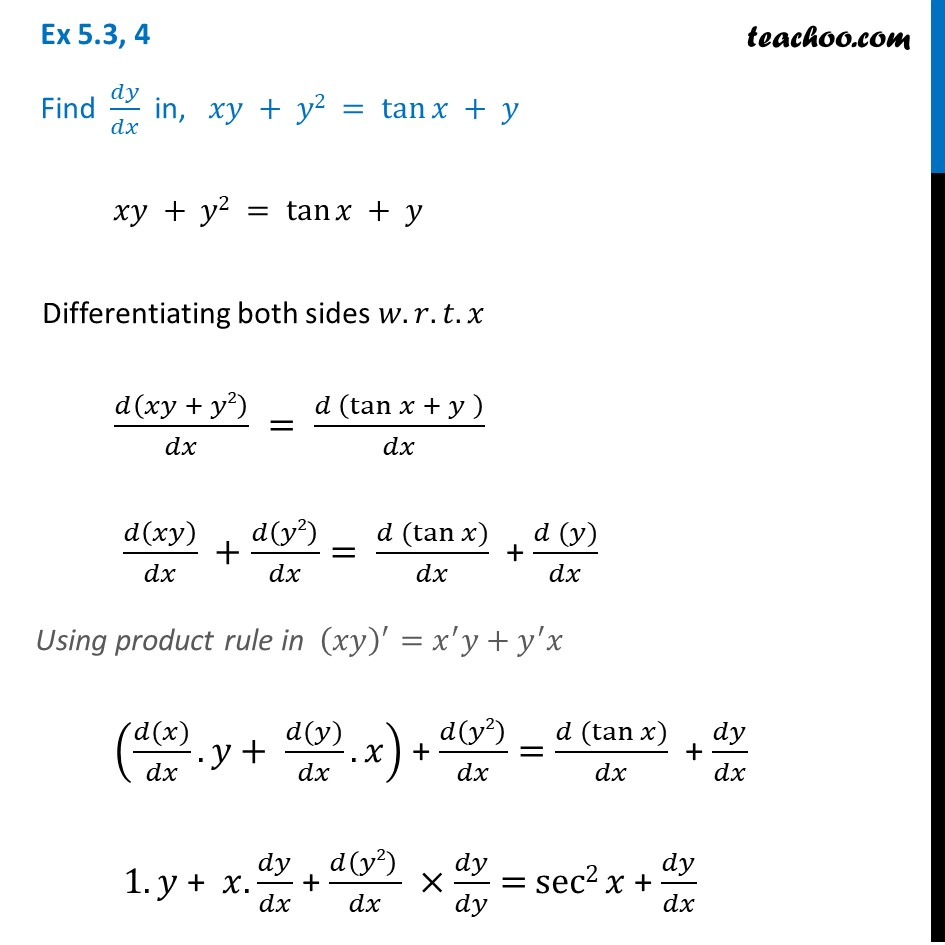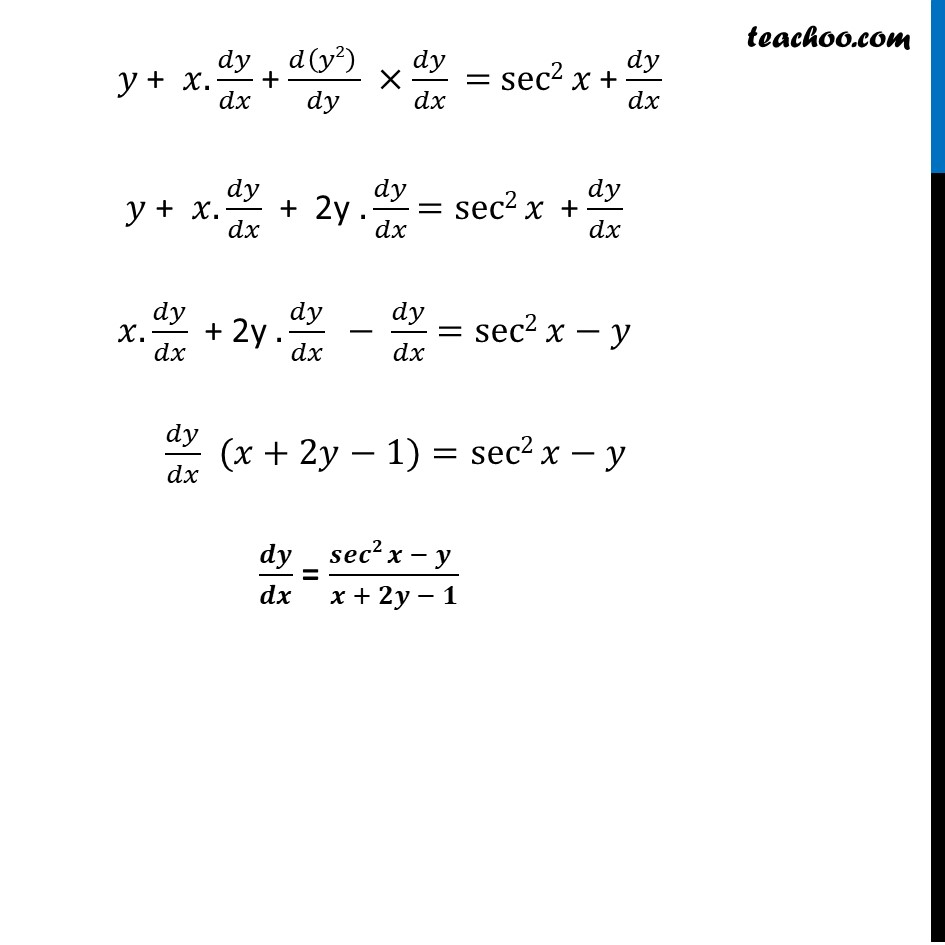Ex 5.3

Chapter 5 Class 12 Continuity and Differentiability
Serial order wiseLearn in your speed, with individual attention - Teachoo Maths 1-on-1 Class

### Transcript

Ex 5.3, 4 Find 𝑑𝑦/𝑑𝑥 in, 𝑥𝑦 + 𝑦2 = tan⁡𝑥 + 𝑦 𝑥𝑦 + 𝑦2 = tan⁡𝑥 + 𝑦 Differentiating both sides 𝑤.𝑟.𝑡.𝑥 𝑑(𝑥𝑦 + 𝑦2)/𝑑𝑥 = (𝑑 (tan⁡𝑥 + 𝑦" " ))/𝑑𝑥 𝑑(𝑥𝑦)/𝑑𝑥 +𝑑(𝑦2)/𝑑𝑥= (𝑑 (tan⁡𝑥))/𝑑𝑥 + (𝑑 (𝑦))/𝑑𝑥 Using product rule in (𝑥𝑦)^′=𝑥^′ 𝑦+𝑦^′ 𝑥 ((𝑑(𝑥))/𝑑𝑥. 𝑦+ (𝑑(𝑦))/𝑑𝑥.𝑥) + 𝑑(𝑦2)/𝑑𝑥= (𝑑 (tan⁡𝑥))/𝑑𝑥 + 𝑑𝑦/𝑑𝑥 1.𝑦 + 𝑥.𝑑𝑦/𝑑𝑥 + (𝑑(𝑦2) )/𝑑𝑥 ×𝑑𝑦/𝑑𝑦= sec2 𝑥 + 𝑑𝑦/𝑑𝑥 𝑦 + 𝑥.𝑑𝑦/𝑑𝑥 + (𝑑(𝑦2) )/𝑑𝑦 ×𝑑𝑦/𝑑𝑥 = sec2 𝑥 + 𝑑𝑦/𝑑𝑥 𝑦 + 𝑥.𝑑𝑦/𝑑𝑥 + 2y .𝑑𝑦/𝑑𝑥 =sec2 𝑥 + 𝑑𝑦/𝑑𝑥 𝑥.𝑑𝑦/𝑑𝑥 + 2y .𝑑𝑦/𝑑𝑥 " "− 𝑑𝑦/𝑑𝑥 =sec2 𝑥−𝑦 𝑑𝑦/𝑑𝑥 (𝑥+2𝑦−1)=sec2 𝑥−𝑦 𝒅𝒚/𝒅𝒙 = (𝒔𝒆𝒄𝟐 𝒙 − 𝒚" " )/(𝒙 + 𝟐𝒚 − 𝟏)